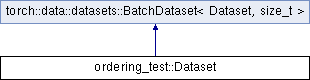Caffe2 - C++ API A deep learning, cross platform ML framework
ordering_test::Dataset Struct Reference
Inheritance diagram for ordering_test::Dataset:## Public Member Functions

Dataset (const Dataset &other)

Dataset (Dataset &&other) noexcept=default

Datasetoperator= (const Dataset &other)=delete

Datasetoperator= (Dataset &&other) noexcept=delete

size_t get_batch (torch::ArrayRef< size_t > indices) override

torch::optional< size_t > size () const override
Returns the size of the dataset, or an empty optional if it is unsized.Public Member Functions inherited from torch::data::datasets::BatchDataset< Dataset, size_t >
virtual size_t get_batch (ArrayRef< size_t >request)=0
Returns a batch of data given an index.

MapDataset< Dataset, TransformType > map (TransformType transform)&
Creates a `MapDataset` that applies the given `transform` to this dataset.

MapDataset< Dataset, TransformType > map (TransformType transform)&&
Creates a `MapDataset` that applies the given `transform` to this dataset.

## Data FieldsPublic Types inherited from torch::data::datasets::BatchDataset< Dataset, size_t >
using SelfType = Dataset

using BatchType = size_t

using BatchRequestType = ArrayRef< size_t >Static Public Attributes inherited from torch::data::datasets::BatchDataset< Dataset, size_t >
static constexpr bool is_stateful

## Detailed Description

Definition at line 1344 of file dataloader.cpp.

The documentation for this struct was generated from the following file: